# RGB Matrix module 20x40 1/5 scan GKGD - error of display of the image

This site uses cookies. By continuing to browse this site, you are agreeing to our Cookie Policy.

• ### Source Code

1. Display_refresh:
3. If Page_counter < 4 Then
4. Incr Page_counter
5. Else
6. Page_counter = 0
7. End If
8. Index_1 = Page_counter * 5
9. Atemp = 0
10. For D_1 = 1 To 10
11. Index_2 = Index_1 + 50
12. If D_1.0 = 1 Then
13. Shift6out Memory_red(index_1) , Memory_green(index_1) , Memory_blue(index_1) , Memory_red(index_2) , Memory_green(index_2) , Memory_blue(index_1)
14. Else
15. Shift6lout Memory_red(index_1) , Memory_green(index_1) , Memory_blue(index_1) , Memory_red(index_2) , Memory_green(index_2) , Memory_blue(index_2)
16. End If
17. If Atemp = 0 Then
18. Index_1 = Index_1 + 25
19. Incr Atemp
20. Else
21. Index_1 = Index_1 - 24
22. Atemp = 0
23. End If
24. Next D_1
25. Pwm1b = 0
26. Waitus 100
27. P_lat = 1 : P_lat = 0
28. P_a = Page_counter.0
29. P_b = Page_counter.1
30. P_c = Page_counter.2
31. Pwm1b = Brightness
32. Trigger = 1
33. Return
Display All
I hope it was my last Copy and Paste error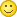• SUPER!!!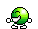I just corrected the blue color
Shift6out Memory_red(index_1) , Memory_green(index_1) , Memory_blue(index_1) , Memory_red(index_2) , Memory_green(index_2) , Memory_blue(index_1)
image_50_1.jpg image_50_2.jpg image_50_3.jpg
I will try to make other fonts and scrolling now
•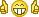Copy & Paste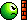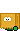Whitch Sofware you use to create Images like image 46_2_true (#193)
Please show some scrolling and other fonts
What happend you overload? (to much letters or to big font)

The post was edited 1 time, last by Pluto25 ().

• Video Test
Works very well!
Sometimes at maximum brightness, there are flashes of LEDs. But it is rare.
Files
• GKGD_image_font.bas

• Font_6x8.bas

• Font_12x16.bas

• Thanks, nice too be helpfull.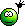Schön das wir helfen konnten.
• Hi
There must be a Pin what selects the Panel ? (Enable or ChipSelect) The OE is only to shut off?

You work with differend ' Set_text_6_8 ' . Isn't it bedder to give it differend names?
Maybe Set_text_6_8v /Set_text_6_8h
• The font is fine. This is a special font that outputs text vertically on the matrix.
Connection of matrixes consecutive
3-GKGD_004.jpg
• Lets have a look to this resulds

### Source Code

1. ' For R_0 = 1 To Dy_num_vertik
2. Index_1 = Page_counter * 5
3. Atemp = 0
4. For D_1 = 1 To 30
5. Index_2 = Index_1 + 50
6. If D_1.0 = 1 Then
7. Shift6out Memory_red(index_1) , Memory_green(index_1) , Memory_blue(index_1) , Memory_red(index_2) , Memory_green(index_2) , Memory_blue(index_2)
8. Else
9. Shift6lout Memory_red(index_1) , Memory_green(index_1) , Memory_blue(index_1) , Memory_red(index_2) , Memory_green(index_2) , Memory_blue(index_2)
10. End If
11. If Atemp = 0 Then
12. Index_1 = Index_1 + 25
13. Incr Atemp
14. Else
15. Index_1 = Index_1 - 24
16. Atemp = 0
17. End If
18. Next D_1
19. ' Next R_0
Display All
Are they always standig in row or could it be that they are lying in row (other code neede)
• Realy? Is G1 on Panel top the same as G1 bottom? Elektrical same ( R=0 Ohm) no shift registers?
So there must be an CS Pin. What exactly does Lat and OE?
• ### Pluto25 wrote:

Realy? Is G1 on Panel top the same as G1 bottom? Elektrical same ( R=0 Ohm) no shift registers?
So there must be an CS Pin. What exactly does Lat and OE?
I drew the Qjang Li matrix diagramGKGD matrices have a slightly different scheme, but the principle is the same
Files
• circuit.pdf

• It could work :

### Source Code

1. Display_refresh:
3. If Page_counter < 4 Then
4. Incr Page_counter
5. Else
6. Page_counter = 0
7. End If
8. For R_0 = 1 To Dy_num_vertik
9. Atemp = R_0 - 1
10. Atemp = Atemp * 100
11. Index_1 = Page_counter * 5
12. Index_1 = Index_1 + Atemp
13. Atemp = 0
14. For D_1 = 1 To Num_matr '10
15. Index_2 = Index_1 + 50
16. If D_1.0 = 1 Then
17. Shift6out Memory_red(index_1) , Memory_green(index_1) , Memory_blue(index_1) , Memory_red(index_2) , Memory_green(index_2) , Memory_blue(index_2)
18. Else
19. Shift6lout Memory_red(index_1) , Memory_green(index_1) , Memory_blue(index_1) , Memory_red(index_2) , Memory_green(index_2) , Memory_blue(index_2)
20. End If
21. If Atemp = 0 Then
22. Index_1 = Index_1 + 25
23. Incr Atemp
24. Else
25. Index_1 = Index_1 - 24
26. Atemp = 0
27. End If
28. Next D_1
29. Next R_0
30. Trigger = 0
31. Pwm1b = 0
32. Waitus 100
33. P_lat = 1 : P_lat = 0
34. P_a = Page_counter.0
35. P_b = Page_counter.1
36. P_c = Page_counter.2
37. Pwm1b = Brightness
38. Trigger = 1
39. Return
Display All
• New

Really works! And everything is just in code.
3-GKGD_005.jpg

What if you connect multiple horizontal matrices?
For D_1 = 1 To Num_matr - does not work
• New

Yes made the test. On 2 and 3 matrix the same image as on 1 matrix